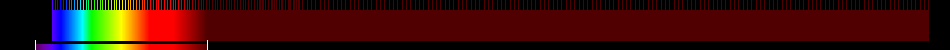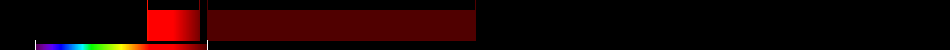# Information for Sensor and Index

## Formula

General Formula $\frac{{\mathrm{NIR}}}{{\mathrm{RED}}+\frac{{b}}{{a}}}$ $\frac{{\mathrm{108}}}{{\mathrm{44}}+\frac{{b}}{{a}}}$ Automatic

## Sensor

Name EnMap Environmental Mapping and Analysis Program 244 420-2450 30-30 0 DLR 2015-00-00 yes

## Index

Name Soil-adjusted vegetation index 2 SAVI2 $\frac{{\mathrm{NIR}}}{{\mathrm{RED}}+\frac{{b}}{{a}}}$ The actual gain (a) and intercept (b) values of the soil line rather than assuming them to be 1 and 0, respectively 640:760,780:1400 Original formula

## References